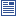Accessibility options:

# Inverse resources

Show me all resources applicable to

### Community Project (1)Mathematical Tools for Physical Sciences and Systems Biology (SOURCE)
A zip file containing the LaTex source files and metatdata for the leaflet - a summary of common mathematical definitions and properties used in the Physical Sciences and Systems Biology contributed to the mathcentre Community Project by Dr Morgiane Richard, University of Aberdeen and reviewed by Mamen Romano and Ian Stansfield, University of Aberdeen.

### Facts & Formulae Leaflets (1)Mathematical Tools for Physical Sciences and Systems Biology
This leaflet is a summary of common mathematical definitions and properties used in the Physical Sciences and Systems Biology contributed to the mathcentre Community Project by Dr Morgiane Richard, University of Aberdeen and reviewed by Mamen Romano and Ian Stansfield, University of Aberdeen.

### Quick Reference (2)Matrix inversion of a 3x3 matrix
Matrices 11: This leaflet explains how to calculate the inverse of a 3x3 matrix. There is an accompanying video tutorial.The inverse of a 2x2 matrix
Matrices 7: This leaflet explains what is meant by the inverse of a 2x2 matrix and shows how to calculate this when it exists. There is an accompanying video tutorial.

### Video (2)Matrix inversion of a 3x3 matrix
Matrices 11: This video tutorial explains how to calculate the inverse of a 3x3 matrix. There is an accompanying help leaflet.The inverse of a 2x2 matrix
Matrices 7: This video tutorial explains what is meant by the inverse of a 2x2 matrix and shows how to calculate this when it exists. There is an accompanying help leaflet.

### Video with captions which require edits (2)Matrix inversion of a 3x3 matrix
Matrices 11: This video tutorial explains how to calculate the inverse of a 3x3 matrix. There is an accompanying help leaflet.The inverse of a 2x2 matrix
Matrices 7: This video tutorial explains what is meant by the inverse of a 2x2 matrix and shows how to calculate this when it exists. There is an accompanying help leaflet.